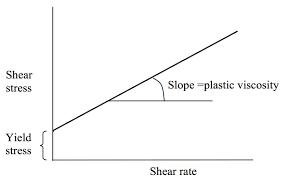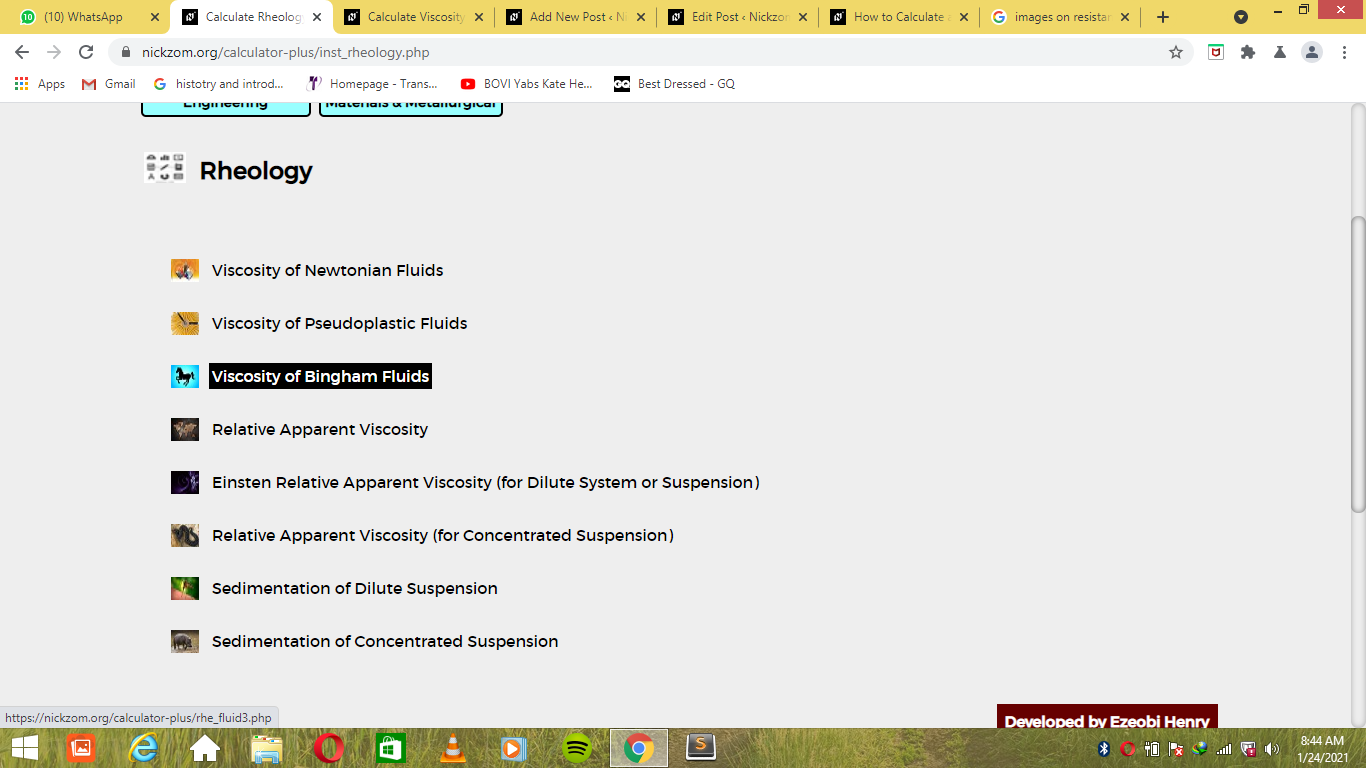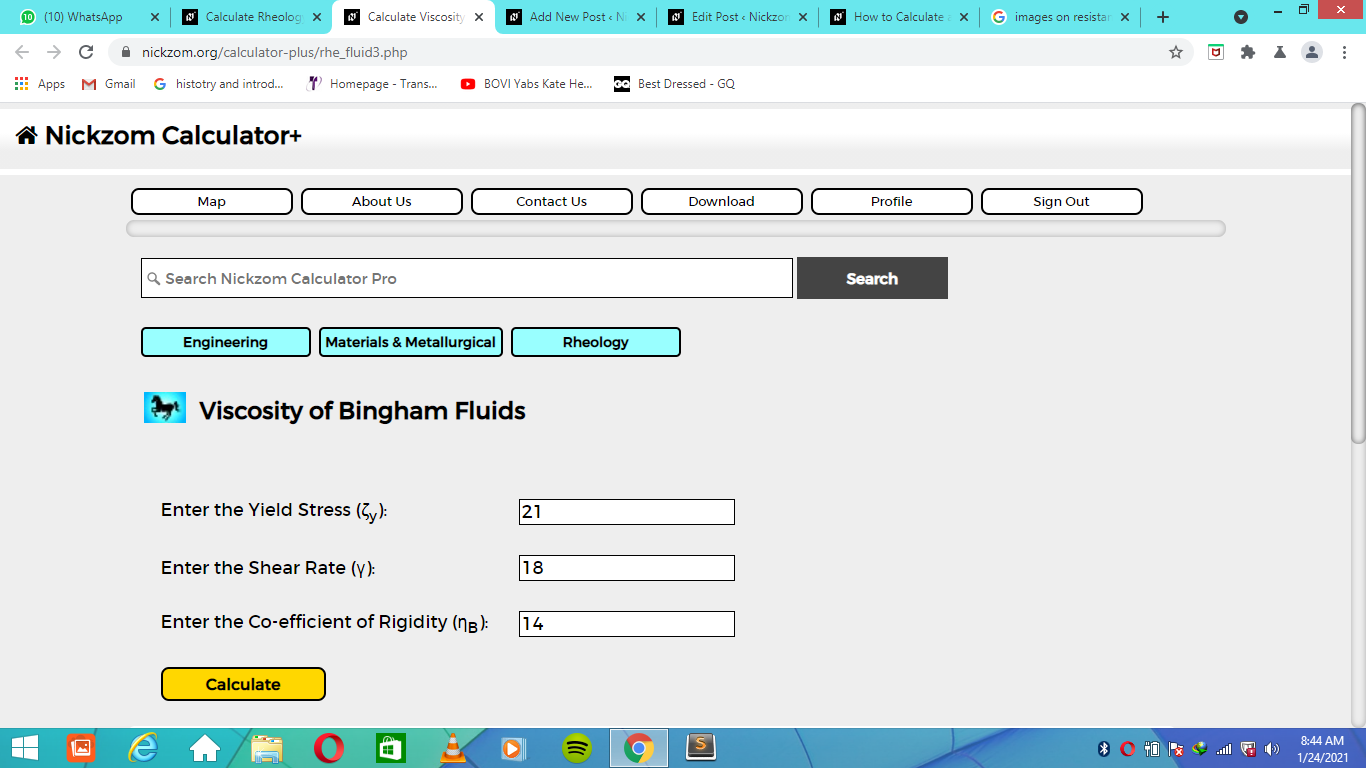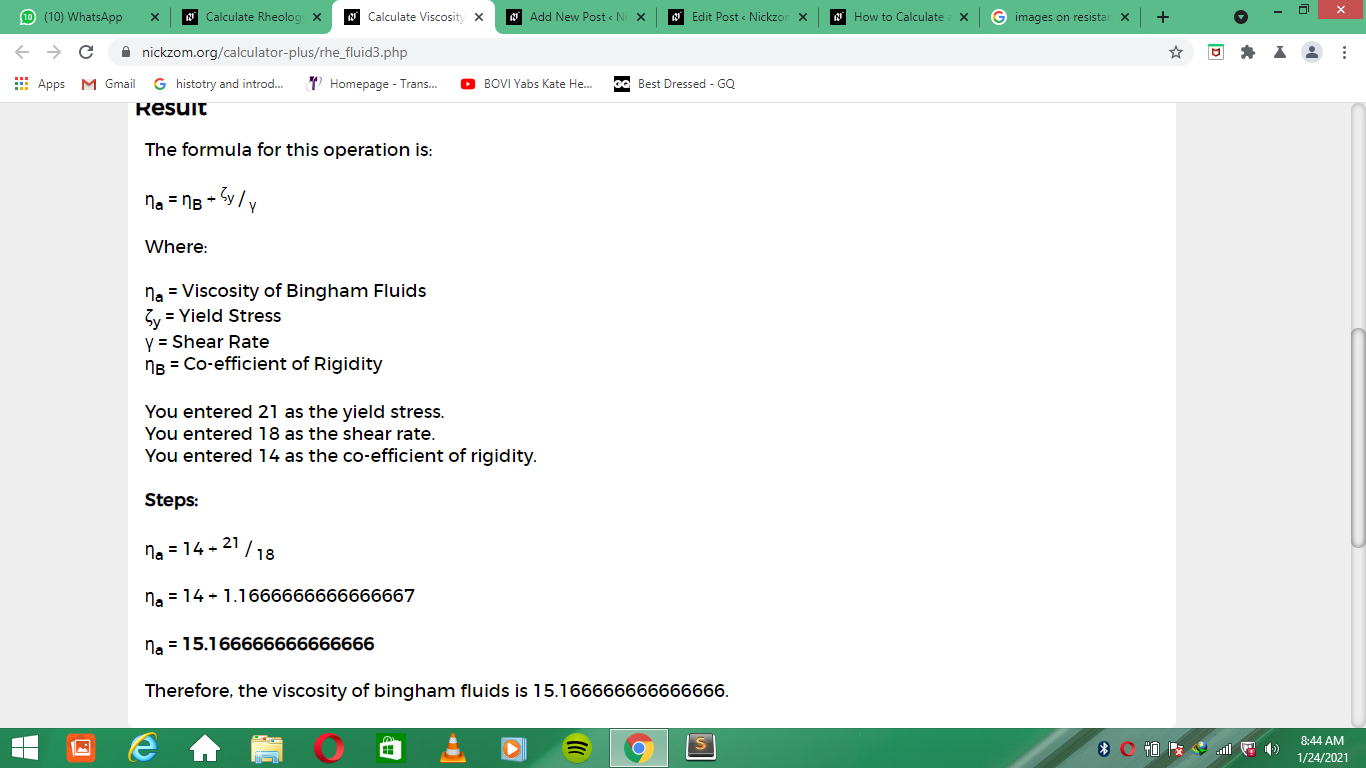# How to Calculate and Solve for Viscosity of Bingham Fluids | RheologyThe image above represents viscosity of bingham fluids.

To compute for viscosity of bingham fluids, three essential parameters are needed and these parameters are Yield Stress (ζy), Shear Rate (γ) and Co-efficient of Rigidity (ηB).

The formula for calculating viscosity of bingham fluids:

ηa = ηB + ζy / γ

Where:

ηa = Viscosity of Bingham Fluids
ζy = Yield Stress
γ = Shear Rate
ηB = Co-efficient of Rigidity

Let’s solve an example;
Find the viscosity of bingham fluids when the yield stress is 21, the shear rate is 18 and co-efficient of rigidity is 14.

This implies that;

ζy = Yield Stress = 21
γ = Shear Rate = 18
ηB = Co-efficient of Rigidity = 14

ηa = ηB + ζy / γ
ηa = 14 + 21 / 18
ηa = 14 + 1.166
ηa = 15.16

Therefore, the viscosity of bingham fluids is 15.16.

Calculating for Yield Stress when the Viscosity of Bingham Fluids, the Shear Rate and the Co-efficient of Rigidity is Given.

ζy = (ηa – ηB) γ

Where;

ζy = Yield Stress
ηa = Viscosity of Bingham Fluids
γ = Shear Rate
ηB = Co-efficient of Rigidity

Let’s solve an example;
Find the yield stress when the viscosity of bingham fluids is 12, the shear rate is 14 and the co-efficient of rigidity is 8.

This implies that;

ηa = Viscosity of Bingham Fluids = 12
γ = Shear Rate = 14
ηB = Co-efficient of Rigidity = 8

ζy = (ηa – ηB) γ
ζy = (12 – 8) 14
ζy = (4) 14
ζy = 56

Therefore, the yield stress is 56.

Calculating for Shear Rate when the Viscosity of Bingham Fluids, the Yield Stress and the Co-efficient of Rigidity is Given.

γ = a – ηB) / ζy

Where;

γ = Shear Rate
ηa = Viscosity of Bingham Fluids
ζy = Yield Stress
ηB = Co-efficient of Rigidity

Let’s solve an example;
Find the shear rate when the viscosity of bingham fluids is 20, the yield stress is 15 and the co-efficient of rigidity is 12.

This implies that;

ηa = Viscosity of Bingham Fluids = 20
ζy = Yield Stress = 15
ηB = Co-efficient of Rigidity = 12

γ = a – ηB) / ζy
γ = (20 – 12) / 15
γ = 8 / 15
γ = 0.53

Therefore, the shear rate is 0.53.

Calculating for Co-efficient of Rigidity when the Viscosity of Bingham Fluids, the Yield Stress and the Shear Rate is Given.

ηB = ηa – (ζy / γ)

Where;

ηB = Co-efficient of Rigidity
ηa = Viscosity of Bingham Fluids
ζy = Yield Stress
γ = Shear Rate

Let’s solve an example;
Find the co-efficient of rigidity when the viscosity of bingham fluids is 14, the yield stress is 10 and the shear rate is 6.

This implies that;

ηa = Viscosity of Bingham Fluids = 14
ζy = Yield Stress = 10
γ = Shear Rate = 6

ηB = ηa – (ζy / γ)
ηB = 14 – (10 / 6)
ηB = 14 -1.667
ηB = 12.33

Therefore, the co-efficient of rigidity is 12.33.

Nickzom Calculator – The Calculator Encyclopedia is capable of calculating the viscosity of bingham fluids.

To get the answer and workings of the viscosity of bingham fluids using the Nickzom Calculator – The Calculator Encyclopedia. First, you need to obtain the app.

You can get this app via any of these means:

To get access to the professional version via web, you need to register and subscribe for NGN 2,000 per annum to have utter access to all functionalities.
You can also try the demo version via https://www.nickzom.org/calculator

Apple (Paid) – https://itunes.apple.com/us/app/nickzom-calculator/id1331162702?mt=8
Once, you have obtained the calculator encyclopedia app, proceed to the Calculator Map, then click on Materials and Metallurgical under Engineering.Now, Click on Rheology under Materials and MetallurgicalNow, Click on Viscosity of Bingham Fluids under RheologyThe screenshot below displays the page or activity to enter your values, to get the answer for the viscosity of bingham fluids according to the respective parameters which is the Yield Stress (ζy), Shear Rate (γ) and Co-efficient of Rigidity (ηB).Now, enter the values appropriately and accordingly for the parameters as required by the Yield Stress (ζy) is 21, Shear Rate (γ) is 18 and Co-efficient of Rigidity (ηB) is 14.Finally, Click on CalculateAs you can see from the screenshot above, Nickzom Calculator– The Calculator Encyclopedia solves for the viscosity of bingham fluids and presents the formula, workings and steps too.